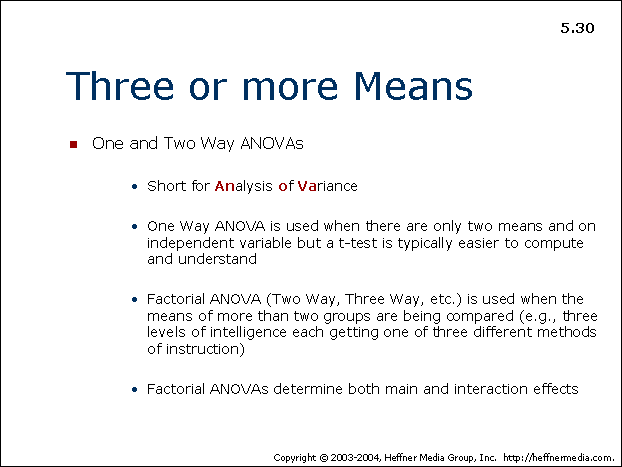advertisement

# 30## Three or more Means

• One and Two Way ANOVAs
• Short for Analysis of Variance
• One Way ANOVA is used when there are only two means and an independent variable but a t-test is typically easier to compute and understand
• Factorial ANOVA (Two Way, Three Way, etc.) is used when the means of more than two groups are being compared (e.g., three levels of intelligence each getting one of three different methods of instructions)
• Factorial ANOVAs determine both main and interaction effects